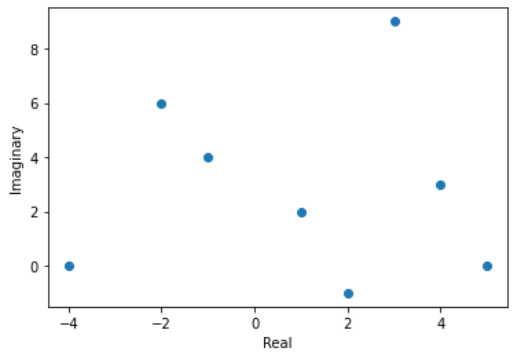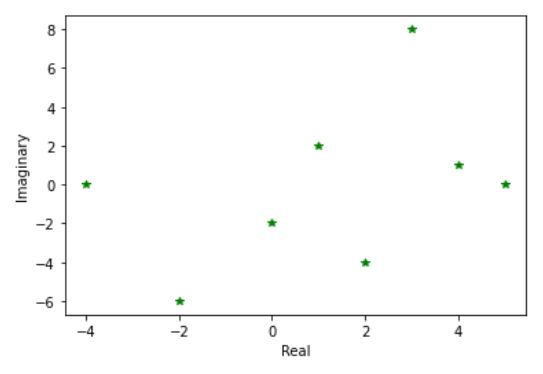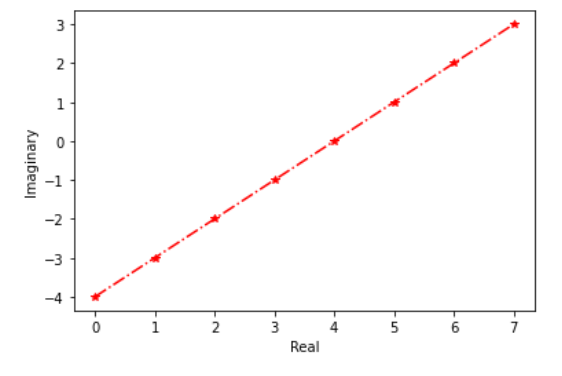Related Articles

# How to plot a complex number in Python using Matplotlib ?

• Last Updated : 03 Jan, 2021

In this article we will learn how to plot complex number in Python using Matplotlib. Let’s discuss some concepts :

• Matplotlib : Matplotlib is an amazing visualization library in Python for 2D plots of arrays. Matplotlib is a multi-platform data visualization library built on NumPy arrays and designed to work with the broader SciPy stack. It was introduced by John Hunter in the year 2002.
• Complex number : A complex number is a number that can be expressed in the form a + bi, where a and b are real numbers, and i represents the imaginary unit, satisfying the equation i2 = −1. Because no real number satisfies this equation, i is called an imaginary number.
• Complex number in Python : An complex number is represented by “ x + yi “. Python converts the real numbers x and y into complex using the function complex(x,y). The real part can be accessed using the function real() and imaginary part can be represented by imag().

Approach:

1. Import libraries.
2. Create data of complex numbers
3. Extract real and imaginary parts from complex numbers data
4. Plot the extracted data.

Examples:

To plot complex numbers, we have to extract its real and imaginary part and to extract and create data, we will use some methods that are explained in below examples :

Example 1 : (Simple plot with complex numbers over real and imaginary data)

## Python3

 `# import library``import` `matplotlib.pyplot as plt`` ` `# create data of complex numbers``data ``=` `[``1``+``2j``, ``-``1``+``4j``, ``4``+``3j``, ``-``4``, ``2``-``1j``, ``3``+``9j``, ``-``2``+``6j``, ``5``]`` ` `# extract real part``x ``=` `[ele.real ``for` `ele ``in` `data]``# extract imaginary part``y ``=` `[ele.imag ``for` `ele ``in` `data]`` ` `# plot the complex numbers``plt.scatter(x, y)``plt.ylabel(``'Imaginary'``)``plt.xlabel(``'Real'``)``plt.show()`

Output :Example 2 : (Using numpy for extracting real and imaginary parts)

## Python3

 `# import libraries``import` `matplotlib.pyplot as plt``import` `numpy as np`` ` `# create data of complex numbers``data ``=` `np.array([``1``+``2j``, ``2``-``4j``, ``-``2j``, ``-``4``, ``4``+``1j``, ``3``+``8j``, ``-``2``-``6j``, ``5``])`` ` `# extract real part using numpy array``x ``=` `data.real``# extract imaginary part using numpy array``y ``=` `data.imag`` ` `# plot the complex numbers``plt.plot(x, y, ``'g*'``)``plt.ylabel(``'Imaginary'``)``plt.xlabel(``'Real'``)``plt.show()`

Output :Example 3 : (Using numpy for creating data of complex numbers and extracting real and imaginary parts)

## Python3

 `# import libraries``import` `matplotlib.pyplot as plt``import` `numpy as np`` ` `# create data of complex numbers using numpy``data ``=` `np.arange(``8``) ``+` `1j``*``np.arange(``-``4``, ``4``)`` ` `# extract real part using numpy``x ``=` `data.real``# extract imaginary part using numpy``y ``=` `data.imag`` ` `# plot the complex numbers``plt.plot(x, y, ``'-.r*'``)``plt.ylabel(``'Imaginary'``)``plt.xlabel(``'Real'``)``plt.show()`

Output :Attention geek! Strengthen your foundations with the Python Programming Foundation Course and learn the basics.

To begin with, your interview preparations Enhance your Data Structures concepts with the Python DS Course. And to begin with your Machine Learning Journey, join the Machine Learning – Basic Level Course

My Personal Notes arrow_drop_up